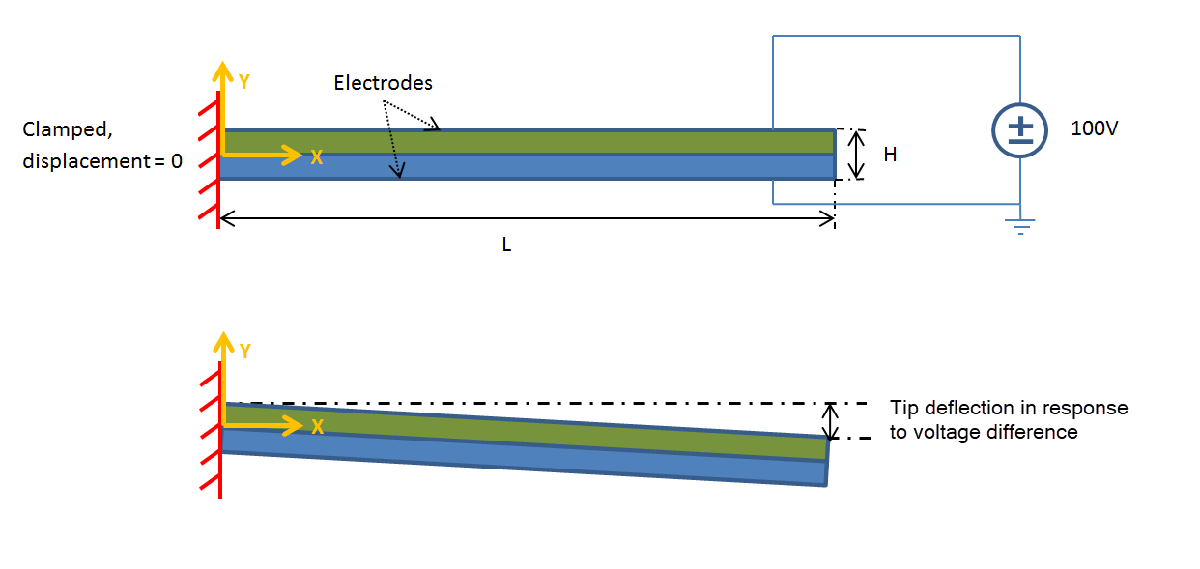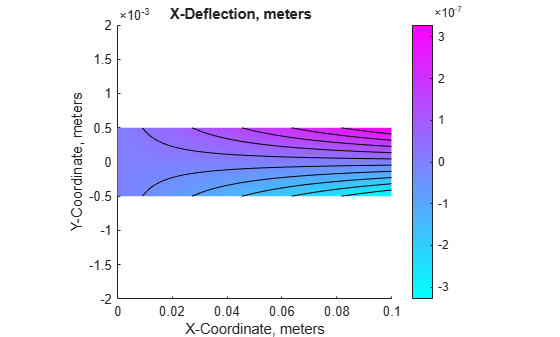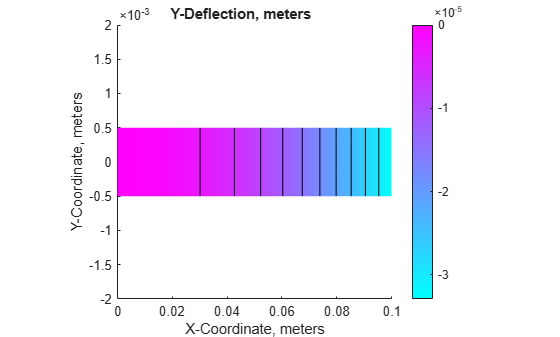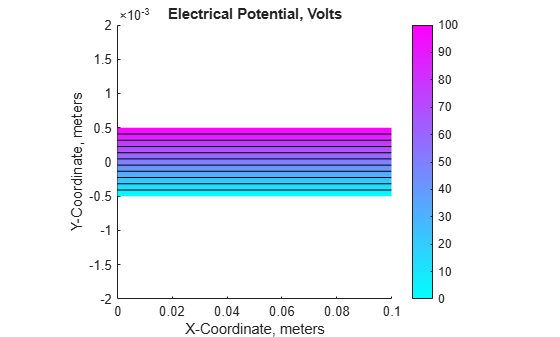Documentation

## Deflection of Piezoelectric Actuator

This example shows how to solve a coupled elasticity-electrostatics problem.

Piezoelectric materials deform when a voltage is applied. Conversely, a voltage is produced when a piezoelectric material is deformed. Analysis of a piezoelectric part requires the solution of a set of coupled partial differential equations with deflections and electrical potential as dependent variables. One of the main objectives of this example is to show how such a system of coupled partial differential equations can be solved using PDE Toolbox.

### PDE For a Piezoelectric Solid

The elastic behavior of the solid is described by the equilibrium equations

`$-\nabla \cdot \sigma =f$`

where $\sigma$ is the stress tensor and $f$ is the body force vector. The electrostatic behavior of the solid is described by Gauss' Law

`$\nabla \cdot D=\rho$`

where $D$ is the electric displacement and $\rho$ is the distributed, free charge. These two PDE systems can be combined into the following single system

`$-\nabla \cdot \left\{\begin{array}{c}\sigma \\ D\end{array}\right\}=\left\{\begin{array}{c}f\\ -\rho \end{array}\right\}$`

In 2D, $\sigma$ has the components ${\sigma }_{11},{\sigma }_{22},$ and ${\sigma }_{12}={\sigma }_{21}$ and $D$ has the components ${D}_{1}$ and ${D}_{2}$.

The constitutive equations for the material define the stress tensor and electric displacement vector in terms of the strain tensor and electric field. For a 2D, orthotropic, piezoelectric material under plane stress conditions these are commonly written as

`$\left\{\begin{array}{c}{\sigma }_{11}\\ {\sigma }_{22}\\ {\sigma }_{12}\\ {D}_{1}\\ {D}_{2}\end{array}\right\}=\left[\begin{array}{ccccc}{C}_{11}& {C}_{12}& & {e}_{11}& {e}_{31}\\ {C}_{12}& {C}_{22}& & {e}_{13}& {e}_{33}\\ & & {G}_{12}& {e}_{14}& {e}_{34}\\ {e}_{11}& {e}_{13}& {e}_{14}& -{\mathcal{E}}_{1}& \\ {e}_{31}& {e}_{33}& {e}_{34}& & -{\mathcal{E}}_{2}\end{array}\right]\left\{\begin{array}{c}{ϵ}_{11}\\ {ϵ}_{22}\\ {\gamma }_{12}\\ -{E}_{1}\\ -{E}_{2}\end{array}\right\}$`

where ${C}_{ij}$ are the elastic coefficients, ${\mathcal{E}}_{i}$ are the electrical permittivities, and ${e}_{ij}$ are the piezoelectric stress coefficients. The piezoelectric stress coefficients are written to conform to conventional notation in piezoelectric materials where the z-direction (3-direction) is aligned with the "poled" direction of the material. For the 2D analysis, we want the poled direction to be aligned with the y-axis.

Finally, the strain vector can be written in terms of the x-displacement, $u$, and y-displacement, $v$ as

`$\left\{\begin{array}{c}{ϵ}_{11}\\ {ϵ}_{22}\\ {\gamma }_{12}\end{array}\right\}=\left\{\begin{array}{c}\frac{\partial u}{\partial x}\\ \frac{\partial v}{\partial y}\\ \frac{\partial u}{\partial y}+\frac{\partial v}{\partial x}\end{array}\right\}$`

and the electric field written in terms of the electrical potential, $\varphi$, as

`$\left\{\begin{array}{c}{E}_{1}\\ {E}_{2}\end{array}\right\}=-\left\{\begin{array}{c}\frac{\partial \varphi }{\partial x}\\ \frac{\partial \varphi }{\partial y}\end{array}\right\}$`

See reference 2, for example, for a more complete description of the piezoelectric equations.

The strain-displacement equations and electric field equations above can be substituted into the constitutive equations to yield a system of equations for the stresses and electrical displacements in terms of displacement and electrical potential derivatives. If the resulting equations are substituted into the PDE system equations, we have a system of equations that involve the divergence of the displacement and electrical potential derivatives. Arranging these equations to match the form required by PDE Toolbox will be the topic for the next section.

### Converting the Equations to PDE Toolbox Form

The PDE Toolbox requires a system of elliptic equations to be expressed in the form

`$-\nabla \cdot \left(c\otimes \nabla u\right)+au=f$`

or in tensor form

`$-\frac{\partial }{\partial {x}_{k}}\left({c}_{ijkl}\frac{\partial {u}_{j}}{\partial {x}_{l}}\right)+{a}_{ij}{u}_{j}={f}_{i}$`

where summation is implied by repeated indices. For the 2D piezoelectric system described above, the PDE Toolbox system vector $u$ is

`$u=\left\{\begin{array}{c}u\\ v\\ \varphi \end{array}\right\}$`

This is an $N=3$ system. The gradient of $u$ is given by

`$\nabla u=\left\{\begin{array}{c}\frac{\partial u}{\partial x}\\ \phantom{\rule{1em}{0ex}}\frac{\partial u}{\partial y}\phantom{\rule{1em}{0ex}}\\ \frac{\partial v}{\partial x}\\ \frac{\partial v}{\partial y}\\ \frac{\partial \varphi }{\partial x}\\ \frac{\partial \varphi }{\partial y}\end{array}\right\}$`

The documentation for the function `assempde` shows that it is convenient to view the tensor ${c}_{ijkl}$ as an $N×N$ matrix of $2×2$ submatrices. The most convenient form for the $c$ input argument for this symmetric, $N=3$ system has 21 rows in $c$ and is described in detail in the PDE Toolbox documentation. It is repeated here for convenience.

`$\left[\begin{array}{cccccc}c\left(1\right)& c\left(2\right)& c\left(4\right)& c\left(6\right)& c\left(11\right)& c\left(13\right)\\ \cdot & c\left(3\right)& c\left(5\right)& c\left(7\right)& c\left(12\right)& c\left(14\right)\\ \cdot & \cdot & c\left(8\right)& c\left(9\right)& c\left(15\right)& c\left(17\right)\\ \cdot & \cdot & \cdot & c\left(10\right)& c\left(16\right)& c\left(18\right)\\ \cdot & \cdot & \cdot & \cdot & c\left(19\right)& c\left(20\right)\\ \cdot & \cdot & \cdot & \cdot & \cdot & c\left(21\right)\end{array}\right]$`

For the purposes of mapping terms from constitutive equations to the form required by PDE Toolbox it is useful to write the $c$ tensor and solution gradient in the following form

`$\left[\begin{array}{cccccc}{c}_{1111}& {c}_{1112}& {c}_{1211}& {c}_{1212}& {c}_{1311}& {c}_{1312}\\ \cdot & {c}_{1122}& {c}_{1221}& {c}_{1222}& {c}_{1321}& {c}_{1322}\\ \cdot & \cdot & {c}_{2211}& {c}_{2212}& {c}_{2311}& {c}_{2312}\\ \cdot & \cdot & \cdot & {c}_{2222}& {c}_{2321}& {c}_{2322}\\ \cdot & \cdot & \cdot & \cdot & {c}_{3311}& {c}_{3312}\\ \cdot & \cdot & \cdot & \cdot & \cdot & {c}_{3322}\end{array}\right]\left\{\begin{array}{c}\frac{\partial u}{\partial x}\\ \phantom{\rule{1em}{0ex}}\frac{\partial u}{\partial y}\phantom{\rule{1em}{0ex}}\\ \frac{\partial v}{\partial x}\\ \frac{\partial v}{\partial y}\\ \frac{\partial \varphi }{\partial x}\\ \frac{\partial \varphi }{\partial y}\end{array}\right\}$`

From this equation the traditional constitutive coefficients can be mapped to the form required for the PDE Toolbox $c$ matrix. Note the minus sign in the equations for electric field. This minus must be incorporated into the $c$ matrix to match the PDE Toolbox convention. This is shown explicitly below.

`$\left[\begin{array}{cccccc}{C}_{11}& \cdot & \cdot & {C}_{12}& {e}_{11}& {e}_{31}\\ \cdot & {G}_{12}& {G}_{12}& \cdot & {e}_{14}& {e}_{34}\\ \cdot & \cdot & {G}_{12}& \cdot & {e}_{14}& {e}_{34}\\ \cdot & \cdot & \cdot & {C}_{22}& {e}_{13}& {e}_{33}\\ \cdot & \cdot & \cdot & \cdot & -{\mathcal{E}}_{1}& \cdot \\ \cdot & \cdot & \cdot & \cdot & \cdot & -{\mathcal{E}}_{2}\end{array}\right]\left\{\begin{array}{c}\frac{\partial u}{\partial x}\\ \phantom{\rule{1em}{0ex}}\frac{\partial u}{\partial y}\phantom{\rule{1em}{0ex}}\\ \frac{\partial v}{\partial x}\\ \frac{\partial v}{\partial y}\\ \frac{\partial \varphi }{\partial x}\\ \frac{\partial \varphi }{\partial y}\end{array}\right\}$`

### Piezoelectric Bimorph Actuator Model

Now that we have defined the equations for a 2D piezoelectric material, we are ready to apply these to a specific model. The model is a two-layer cantilever beam that has been extensively studied (e.g. refs 1 and 2). It is defined as a "bimorph" because although both layers are made of the same Polyvinylidene Fluoride (PVDF) material, in the top layer the polarization direction points down (minus y direction) and in the bottom layer, it points up. A schematic of the cantilever beam is shown in the figure below.This figure is not to scale; the actual thickness/length ratio is 100 so the beam is very slender. When a voltage is applied between the lower and upper surfaces of the beam, it deflects in the y-direction; one layer shortens and the other layer lengthens. Devices of this type can be designed to provide the required motion or force for different applications.

### Create a PDE Model with three dependent variables

The first step in solving a PDE problem is to create a PDE Model. This is a container that holds the number of equations, geometry, mesh, and boundary conditions for your PDE. The equations of linear elasticity have three components, so the number of equations in this model is three.

```N = 3; model = createpde(N);```

### Geometry Creation

The simple two-layer geometry of the beam can be created by defining the sum of two rectangles.

```L = 100e-3; % beam length in meters H = 1e-3; % overall height of the beam H2 = H/2; % height of each layer in meters```

The two lines below contain the columns of the geometry description matrix (GDM) for the two rectangular layers. The GDM is the first input argument to decsg and describes the basic geometric entities in the model.

```topLayer = [3 4 0 L L 0 0 0 H2 H2]; bottomLayer = [3 4 0 L L 0 -H2 -H2 0 0]; gdm = [topLayer; bottomLayer]'; g = decsg(gdm, 'R1+R2', ['R1'; 'R2']');```

Create a geometry entity and append to the PDE model.

```geometryFromEdges(model,g); figure; pdegplot(model, 'EdgeLabels', 'on', 'FaceLabels', 'on'); xlabel('X-coordinate, meters') ylabel('Y-coordinate, meters') axis([-.1*L, 1.1*L, -4*H2, 4*H2]) axis square```### Material Properties and Coefficient Specification

The material in both layers of the beam is Polyvinylidene Fluoride (PVDF), a thermoplastic polymer with piezoelectric behavior.

```E = 2.0e9; % Elastic modulus, N/m^2 NU = 0.29; % Poisson's ratio G = 0.775e9; % Shear modulus, N/m^2 d31 = 2.2e-11; % Piezoelectric strain coefficients, C/N d33 = -3.0e-11;```

Specify relative electrical permittivity of the material at constant stress.

`relPermittivity = 12;`

Specify electrical permittivity of vacuum.

```permittivityFreeSpace = 8.854187817620e-12; % F/m C11 = E/(1-NU^2); C12 = NU*C11; c2d = [C11 C12 0; C12 C11 0; 0 0 G]; pzeD = [0 d31; 0 d33; 0 0];```

The piezoelectric strain coefficients for PVDF are given above but the constitutive relations in the finite element formulation require the piezoelectric stress coefficients. These are calculated on the next line (for details see, for example, reference 2).

```pzeE = c2d*pzeD; D_const_stress = [relPermittivity 0; 0 relPermittivity]*permittivityFreeSpace;```

Convert dielectric matrix from constant stress to constant strain

`D_const_strain = D_const_stress - pzeD'*pzeE;`

As discussed above, it is convenient to view the 21 coefficients required by assempde as a 3 x 3 array of 2 x 2 submatrices. The cij matrices defined below are the 2 x 2 submatrices in the upper triangle of this array.

```c11 = [c2d(1,1) c2d(1,3) c2d(3,3)]; c12 = [c2d(1,3) c2d(1,2); c2d(3,3) c2d(2,3)]; c22 = [c2d(3,3) c2d(2,3) c2d(2,2)]; c13 = [pzeE(1,1) pzeE(1,2); pzeE(3,1) pzeE(3,2)]; c23 = [pzeE(3,1) pzeE(3,2); pzeE(2,1) pzeE(2,2)]; c33 = [D_const_strain(1,1) D_const_strain(2,1) D_const_strain(2,2)]; ctop = [c11(:); c12(:); c22(:); -c13(:); -c23(:); -c33(:)]; cbot = [c11(:); c12(:); c22(:); c13(:); c23(:); -c33(:)]; f = [0 0 0]'; specifyCoefficients(model, 'm', 0,'d', 0,'c', ctop, 'a', 0, 'f', f,'Face',2); specifyCoefficients(model, 'm', 0,'d', 0,'c', cbot, 'a', 0, 'f', f,'Face',1);```

### Boundary Condition Definition

For this example, the top geometry edge (edge 1) has the voltage prescribed as 100 volts. The bottom geometry edge (edge 2) has the voltage prescribed as 0 volts (i.e. grounded). The left geometry edge (edges 6 and 7) have the u and v displacements equal zero (i.e. clamped). The stress and charge are zero on the right geometry edge (i.e. q = 0).

`V = 100;`

Set the voltage (solution component 3) on the top edge to V.

```voltTop = applyBoundaryCondition(model,'mixed','Edge',1,... 'u',V,... 'EquationIndex',3);```

Set the voltage (solution component 3) on the bottom edge to zero.

```voltBot = applyBoundaryCondition(model,'mixed','Edge',2,... 'u',0,... 'EquationIndex',3);```

Set the x and y displacements (solution components 1 and 2) on the left end (geometry edges 6 and 7) to zero.

```clampLeft = applyBoundaryCondition(model,'mixed','Edge',6:7,... 'u',[0 0],... 'EquationIndex',1:2);```

### Mesh Generation

We need a relatively fine mesh to accurately model the bending of the beam.

```hmax = 5e-04; msh = generateMesh(model,'Hmax',hmax,... 'GeometricOrder','quadratic',... 'MesherVersion','R2013a');```

### Finite Element Solution

`result = solvepde(model);`

Extract the `NodalSolution` property from the result, this has the x-deflection in column 1, the y-deflection in column 2, and the electrical potential in column 3. Find the minimum y-deflection, and plot the solution components.

```rs = result.NodalSolution; feTipDeflection = min(rs(:,2)); fprintf('Finite element tip deflection is: %12.4e\n', feTipDeflection);```
```Finite element tip deflection is: -3.2900e-05 ```
```varsToPlot = char('X-Deflection, meters', 'Y-Deflection, meters', ... 'Electrical Potential, Volts'); for i = 1:size(varsToPlot,1) figure; pdeplot(model, 'XYData', rs(:,i), 'Contour', 'on'); title(varsToPlot(i,:)) % scale the axes to make it easier to view the contours axis([0, L, -4*H2, 4*H2]) xlabel('X-Coordinate, meters')```
``` ylabel('Y-Coordinate, meters') axis square end```### Analytical Solution

A simple, approximate, analytical solution was obtained for this problem in reference 1.

```tipDeflection = -3*d31*V*L^2/(8*H2^2); fprintf('Analytical tip deflection is: %12.4e\n', tipDeflection);```
```Analytical tip deflection is: -3.3000e-05 ```

### Summary

The color contour plots of x-deflection and y-deflection show the standard behavior of the classical cantilever beam solution. The linear distribution of voltage through the thickness of the beam is as expected. There is good agreement between the PDE Toolbox finite element solution and the analytical solution from reference 1.

Although this example shows a very specific coupled elasticity-electrostatic model, the general approach here can be used for many other systems of coupled PDEs. The key to applying PDE Toolbox to these types of coupled systems is the systematic, multi-step coefficient mapping procedure described above.

### References

1. Hwang, W. S.; Park, H. C; Finite Element Modeling of Piezoelectric Sensors and Actuators. AIAA Journal, 31(5), pp 930-937, 1993.

2. Pieford, V; Finite Element Modeling of Piezoelectric Active Structures. PhD Thesis, Universite Libre De Bruxelles, 2001.

##### SupportGet trial now